# Precalculus : Find the Roots of Complex Numbers

## Example Questions

### Example Question #1 : Find The Roots Of Complex Numbers

Evaluate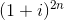, whereis a natural number andis the complex number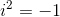.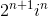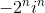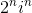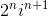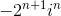Explanation:

Note that,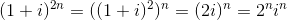### Example Question #2 : Find The Roots Of Complex Numbers

What is the  length of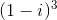?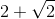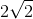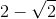Explanation:

We have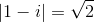.

Hence,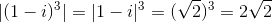.

### Example Question #3 : Find The Roots Of Complex Numbers

Solve for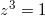(there may be more than one solution).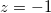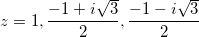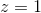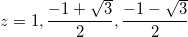Explanation:

Solving that equation is equivalent to solving the roots of the polynomial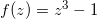.

Clearly, one of roots is 1.

Thus, we can factor the polynomial as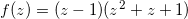so that we solve for the roots of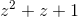.

Using the quadratic equation, we solve for roots, which are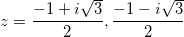.

This means the solutions toare### Example Question #4 : Find The Roots Of Complex Numbers

Recall that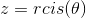is just shorthand for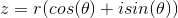when dealing with complex numbers in polar form.

### Express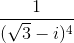in polar form.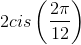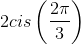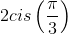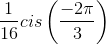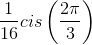Explanation:

First we recognize that we are trying to solve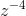where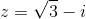.

Then we want to convertinto polar form using,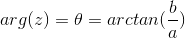and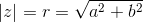.

Then since De Moivre's theorem states,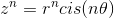ifis an integer, we can say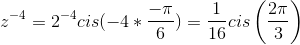.

### All Precalculus Resources